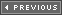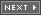### The Evil Head of Friction

The next topic of discussion is friction. Friction is any force that retards or consumes energy from another system. For example, automobiles use internal combustion to operate; however, a whopping 30–40 percent of the energy that is produced is eaten up by thermal conversion or mechanical friction. On the other hand, a bicycle is about 80–90 percent efficient and is probably the most efficient mode of transportation in existence.

#### Basic Friction Concepts

e is basically resistance in the opposite direction of motion and hence can be modeled with a force usually referred to as the frictional force. Take a look at Figure 13.17; it depicts the standard frictional model of a mass m on a flat plane.

##### Figure 13.17. Basic friction model.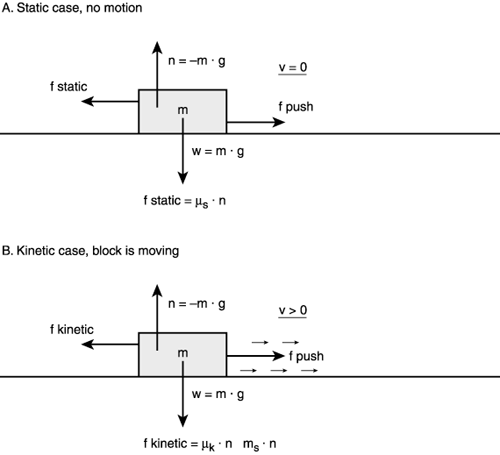If you try to push the mass in a direction parallel to the plane you will encounter a resistance or frictional force that pushes back against you. This force is defined mathematically as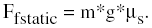where m is the mass of the object, g is the gravitational constant (9.8 m/s2) and _s is the static frictional coefficient of the system that depends on the conditions and materials of the mass and the plane. If the force F you apply to the object is greater than Ff, then the object will begin to move. Once the object is in motion its frictional coefficient usually decreases to another value, which is referred to as the coefficient of kinetic friction, mk.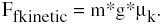When you release the force, the object slowly decelerates and comes to rest because friction is always present.

To model friction on a flat surface all you need do is apply a constant negative velocity to all your objects that is proportional to the friction that you want. Mathematically, this is

```Velocity New = Velocity Old - friction.
```

The result is objects that slow down at a constant rate once you stop moving them. Of course, you have to watch out for letting the sign of the velocity go negative or in the other direction, but that's just a detail. Here's an example of an object that is moved to the right with an initial velocity of 16 pixels per frame and then slowed down at a rate of 1 pixel per frame due to virtual friction:

```int x_pos      = 0,  // starting position
x_velocity = 16, // starting velocity
friction   = -1; // frictional value

// move object until velocity <= 0
while(x_velocity > 0)
{
// move object
x_pos+=x_velocity;
// apply friction
x_velocity+=friction;
}  // end while
```

The first thing you should notice is how similar the model for friction is to gravity. They are almost identical. The truth is that both gravitational forces and frictional forces act in the same way. In fact, all forces in the universe can be modeled in the exact same way. Also, you can apply as many frictional forces to an object as you want. Just sum them up.

As an example of friction I have written a little air hockey demo named DEMO13_5.CPP|EXE (16-bit version, DEMO13_5_16B.CPP|EXE), shown in Figure 13.18. The program lets you fire a hockey puck on a virtual air hockey table in a random direction every time you press the spacebar. The puck then bounces around off the borders of the table until it comes to rest due to friction. If you want to change the frictional coefficient of the table, use the arrow keys. See if you can add a paddle and a computer controlled opponent to the simulation!

##### Figure 13.18. The hockey demo.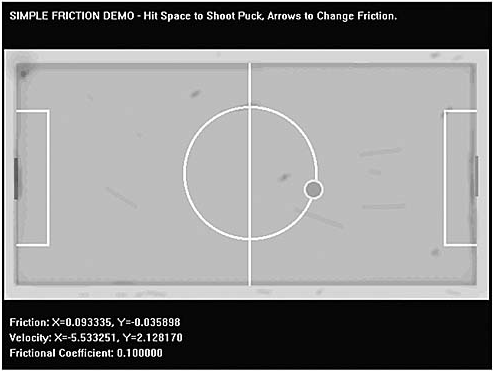#### Friction on an Inclined Plane (Advanced)

That wasn't too bad huh? The bottom line is that friction can be modeled as a simple resistive force or a negative velocity on an object each cycle. However, I want to show you the math and derivation of friction on an inclined plane since this will give you the tools you need to analyze much more complex problems. Be warned, though: I'm going to use a lot of vectors, so if you're still rusty or having trouble then take a look back when I talked about them in Chapter 8, "Vector Rasterization and 2D Transformations," or pick up a good linear algebra book.

Figure 13.19 shows the problem we're trying to solve. Basically, there is a mass m on an inclined plane. The plane has frictional coefficients ms and mk for the static and kinetic (moving) cases respectively. The first thing we need to do is write the formulas that describe the mass in its equilibrium position, that is, not moving. In this case, the sum of the forces in the x-axis are 0 and the sum of the forces in the y-axis are 0.

##### Figure 13.19. The inclined plane problem.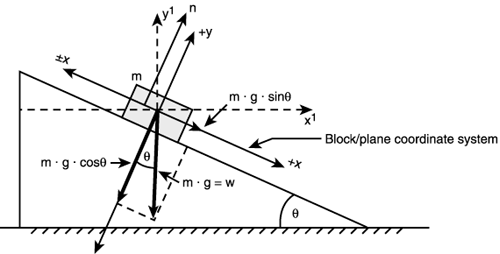To begin the derivation we must first touch on a new concept called the normal force. This is nothing more than the force that the inclined plane pushes the object back with, or in other words, if you weigh 200 lbs., then there is a normal force of –200 lbs. pushing back (due to the surface tension of ground you're standing on) at you. We usually refer to the normal force as h, and it is equal in magnitude to

```h = m*g.
```

Interesting huh? But if you lay a coordinate system down, then the gravity force must be opposite the normal force, or

```h - m*g = 0.
```

This is why everything doesn't get sucked into the ground. Okay, now that we know that, let's derive the motion equations of this block mass. First, we lay down an x,y coordinate system on the incline plane with +X parallel to the plane and in the downward sliding direction; this helps the math. Then we write the equilibrium equations for the x and y axes. For the x-axis we know that the component of gravity pushing the block is

```force due to gravity = m*g*sin q.
```

And the force due to friction holding the block from sliding is

```force due to friction = -h* ms.
```

The negative sign is because the force acts in the opposite direction. When the object isn't sliding we know that the sum of these forces are equal to 0. Mathematically, we have

```force due to gravity + force due to friction = 0
```

Or, the sum of forces in the x-axis is

```S Fx = m*g*sin q - h*ms = 0.
```

MATH

Note that I use sine and cosine to resolve the x,y components of the force. I'm basically just breaking the force vectors into components, nothing more.

We have to do the same for the y-axis, but this is fairly easy because the only forces are the weight of gravity and the normal force:

```S Fy = h - m*g*cos q
```

All right, so all together we have

```S Fx = m*g*sin q - h*ms = 0.
S Fy = h - m*g*cos q = 0.
```

But, what is h? From S Fy, we once again see that:

```h - m*g*cos q = 0.
```

Hence,

```h = m*g*cos q,
```

therefore we can write:

```S Fx = m*g*sin q - (m*g*cos q)*ms = 0.
```

This is what we need. From it we can derive the following results:

```m*g*sin q = (m*g*cos q)*ms

qs = (m*g*sin q)/(m*g*cos q) = tan q
```

Or canceling out the m*g and replacing sin/cos by tan,

```qcritical = tan-1 ms
```

Now listen carefully. This tells us that there is an angle called the critical angle (qcritical) at which the mass starts to slide. It is equal to the inverse tangent of the static coefficient of friction. If we didn't know the frictional coefficient of a mass and some incline plane, we could find it this way by tilting the plane until the mass starts to move. But this doesn't help us with the x-axis, or does it? The equation tells us that the mass won't slide until the angle qcritical is reached. When it is reached the mass will slide governed by:

```S Fx = m*g*sin q - (m*g*cos q)*ms
```

Well, almost… There is one detail. When the mass starts to slide, the difference is m*g*sin q – (m*g*cos q)*_s > 0, but in addition we need to change the frictional coefficient to _k (the coefficient of kinetic friction) to be totally correct!

```Fx = m*g*sin q - (m*g*cos q)*mk
```

TRICK

You can just average mk and ms and use that value in all the calculations. Because you're making video games and not real simulations, it doesn't matter if you oversimplify the two frictional cases into one, but if you want to be correct, you should use both frictional constants at the appropriate times.

With all that in mind let's compute the final force along the x-axis. We know that F=m*a, therefore:

```Fx = m*a = m*g*sin q - (m*g*cos q)*mk
```

And dividing by m we get

```a = g*sin q - (g*cos q)*mk
a = g*(sin q - qk*cos q)
```

You can use this exact model to move the block mass, that is, each cycle you can increase the velocity of the block in the positive X-direction by g*(sin qmk*cos q). There's only one problem: This solution is in our rotated coordinate system! There's a trick to getting around this: You know the angle of the plane, and hence you can figure out a vector along the downward angle of the plane:

```xplane = cos q
yplane = -sin q

Slide_Vector = (cos q, -sin q)
```

The minus sign is on the Y-component because we know it's in the –Y direction. With this vector we can then move the object in the correct direction each cycle—this is a hack, but it works. Here's the code to perform the translation and velocity tracking:

```// Inputs
float x_pos      = SX, // starting point of mass on plane
y_pos      = SY,
y_velocity = 0,  // initial y velocity
x_velocity = 0,  // initial x velocity
x_plane    = 0,  // sliding vector
y_plane    = 0,
gravity    = 1,  // do want to fall too fast
velocity   = INITIAL_VEL, // whatever

// must be in radians and it must be greater
// than the critical angle
angle      = PLANE_ANGLE, // compute velocities in x,y

frictionk   = 0.1; // frictional value

// compute trajectory vector
x_plane = cos(angle);
y_plane = sin(angle); // no negative since +y is down

// do slide loop until object hits
// bottom of screen at SCREEN_BOTTOM
while(y_pos < SCREEN_BOTTOM)
{
// update position
x_pos+=x_velocity;
y_pos+=y_velocity;

// update velocity
x_vel+=x_plane*gravity*(sin(angle) - frictionk *cos(angle));
y_vel+=y_plane*gravity*(sin(angle) - frictionk *cos(angle));

}  // end while
```

The point of physics modeling sometimes is just to understand what the underlying physics are so you can model them in a somewhat convincing manner. In the case of the incline plane, basically all that math just boiled down to the concept that acceleration is a function of the angle (we knew this from common sense). However, in Volume II of the book I'm going to cover much more realistic physics using numerical integration, and in those cases, you need to know the real models and real forces on everything.

 I l@ve RuBoard